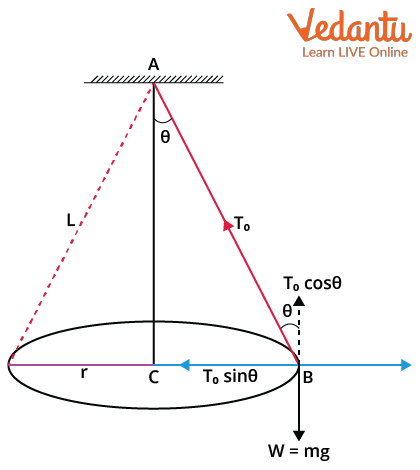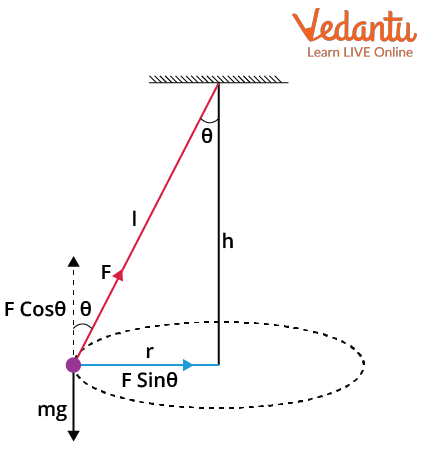Courses
Courses for Kids
Free study material
Free LIVE classes
More

# Conical Pendulum - JEE Important Topic## Conical Pendulum: Introduction

Last updated date: 20th Mar 2023
Total views: 77.4k
Views today: 1.55k

A conical pendulum is a simple pendulum in which the string moves along the surface of a cone and the point object performs a horizontal circular motion. It is similar to a simple pendulum with the difference that the mass or bob, instead of moving back and forth, swings around in a horizontal circle. In a conical pendulum, the bob moves at a constant speed in a circle with the string tracing out a cone.

The conical pendulum has had a long history, and it was first studied by Robert Hooke. He was a famous English scientist who studied the conical pendulum around 1660 as a model for the orbital motion of the planets. Later, inspired by Hooke’s work on conical pendulums, Christian Huygens calculated its time period in 1673. He calculated the time period of the conical pendulum using the concept of centrifugal force which he introduced then. In this article, we will discuss the time period and tension of a conical pendulum

## Time Period of a Conical Pendulum

Let’s derive an expression for the period of a conical pendulum.Schematic Diagram of a Conical Pendulum

Where,

c = Centre of the circle

r = Radius of the circle

L = Length of the string

m = Mass of the bob

$\omega$ = Weight of the bob ($\omega=mg$, g= acceleration due to gravity)

To = Tension in the string

$\theta$ = Semi-vertical angle ( The angle made by the string of the conical pendulum with the vertical)

The two forces acting on the bob are

1. Due to weight (mg) - vertically downward

2. Due to tension (To) - Directed along the string towards point A

For equilibrium of the bob, the tension is resolved into two components

1. $T_{o} \cos \theta$ is acting along the vertical direction.

2. $T_{o} \sin \theta$ is acting along the horizontal direction.

The component $T_{o} \cos \theta$ is balanced by weight mg

$T_{o} \cos \theta=mg$ ….(1)

The component $T_{o} \sin \theta$ is balanced by necessary centrifugal force.

The component $T_{o} \sin \theta$ provides the necessary centripetal force which is required for circular motion.

$T_{o} \sin \theta=\dfrac{m v^{2}}{r}=m r \omega^{2}$ ….(2)

Dividing equation (2) by equation (1), we get

\begin{align}&\dfrac{T_{o} \sin \theta}{T_{o} \cos \theta}=\dfrac{m r \omega^{2}}{m g} \\ &\dfrac{\sin \theta}{\cos \theta}=\dfrac{r \omega^{2}}{g} \\ &\omega^{2}=\dfrac{g}{r} \dfrac{\sin \theta}{\cos \theta}......(3) \end{align}

Now in the $\triangle B A C$,

\begin{align} &\sin \theta=\dfrac{r}{L} \\ &r=L \sin \theta….(4) \end{align}

Put the value of r from equation (4) into the equation (3),

\begin{align} \omega^{2} &=\dfrac{g}{L \sin \theta} \dfrac{\sin \theta}{\operatorname{con} \theta} \\ \omega^{2} &=\dfrac{g}{L \cos \theta}....(5) \end{align}

We already know that $\omega=\dfrac{\theta}{t}$ and $T=\dfrac{2 \pi r}{v}$

From

\begin{align} &\nu=r \omega \\ &\dfrac{\nu}{r}=\omega \end{align}

Substituting this into the equation of T, we get

\begin{align} &T=\dfrac{2 \pi}{\omega} \\ &\omega=\dfrac{2 \pi}{T} \end{align}

Then the equation (5) will become

$\dfrac{2 \pi}{T}=\sqrt{\dfrac{g}{L \cos \theta}}$

Rearranging the equation, we will get

$T=2 \pi \sqrt{\dfrac{L \cos \theta}{g}}$

This is the equation for the time period (T) of the conical pendulum.

### Notes on Time Period of Conical Pendulum

• The equation for the time period (T) of the conical pendulum,

$T=2 \pi \sqrt{\dfrac{L \cos \theta}{g}}$

• The time period of a conical pendulum is directly proportional to the square root of the length of the string.

$\mathrm{T} \propto \sqrt{L}$

• The time period of a conical pendulum is directly proportional to the square root of the cosine of the semi-vertical angle.

$T \propto \sqrt{\cos \theta}$

• The time period of a conical pendulum is inversely proportional to the square root of the acceleration due to the gravity of that place.

$\mathrm{T} \propto \dfrac{1}{\sqrt{g}}$

• The time period of a conical pendulum is independent of the mass of the bob.

## Tension in the String of Conical Pendulum

Derive an expression for the tension in the string of the conical pendulum.Schematic Diagram of a Conical Pendulum

The two components of the tension (To) are given by

$T_{o} \cos \theta=m g$ ….(1)

$T_{o} \sin \theta=\dfrac{m v^{2}}{r}$ ….(2)

Dividing the equation (2) by equation (1),

$\dfrac{T_{o} \sin \theta}{T_{o} \cos \theta}=\dfrac{\dfrac{m v^{2}} { r}}{m g}$

$\tan \theta=\dfrac{v^{2}}{r g}$ ….(3)

Squaring the equations (1), and (2). Then adding them,

\begin{align} &T_{o}^{2} \sin ^{2} \theta+T_{o}^{2} \cos ^{2} \theta=\left(\dfrac{m v^{2}}{r}\right)^{2}+(m g)^{2} \\ &T_{o}^{2}\left(\sin ^{2} \theta+\cos ^{2} \theta\right)=m^{2}\left(\dfrac{v^{2}}{r}\right)^{2}+m^{2} g^{2} \end{align}

Where we already know that $\sin ^{2} \theta+\cos ^{2} \theta=1$. Applying that in the equation and

\begin{align} &T_{o}^{2}=m^{2}\left(\left(\dfrac{v^{2}}{r}\right)^{2}+g^{2}\right) \\ &T_{o}=m \sqrt{\left(\dfrac{v^{2}}{r}\right)^{2}+g^{2}}...(4)\end{align}

From equation (3),

\begin{align} &\tan \theta=\dfrac{v^{2}}{r g}=\dfrac{r}{h} \\ &v^{2}=\dfrac{r^{2} g}{h}...(5) \end{align}

Substituting equation (5) in equation (4), we get

\begin{align} &T_{o}=m \sqrt{\left(\dfrac{r^{2} g}{h} \times \dfrac{1}{r}\right)^{2}+g^{2}} \\ &T_{o}=m \sqrt{\left(\dfrac{r g}{h}\right)^{2}+g^{2}} \\ &T_{o}=m \sqrt{\dfrac{r^{2} g^{2}}{h^{2}}+g^{2}} \\ &T_{o}=m \sqrt{g^{2}\left(\dfrac{r^{2}}{h^{2}}+1\right)} \\ &T_{o}=m g \sqrt{1+\left(\dfrac{r}{h}\right)^{2}} \end{align}

This is the expression for the tension in the string of the conical pendulum.

## Simple Pendulum

A simple is a special case of a conical pendulum. In the case of the simple pendulum, the semi-vertical angle ($\theta$) is zero. So, the movement of the bob will be back and forth.

The time period of a conical pendulum can be written as

$T=2 \pi \sqrt{\dfrac{L \cos \theta}{g}}$

Where $\theta = 0$ in the case of a simple pendulum, then the equation will become

\begin{align} &T=2 \pi \sqrt{\dfrac{L \cos 0^\circ}{g}} \\ &T=2 \pi \sqrt{\dfrac{L}{g}} \end{align}

This is the equation for the time period of a simple pendulum.

Here,

T = Time period of the simple pendulum

L = Length of the pendulum string

g = Acceleration due to gravity

## Numerical Examples

Example 1: A cord of length 5m is fixed at one end and to its other end weight is attached, which describes a horizontal circle of radius 1.2m. Find the time period of the weight and the speed of the weight in the circular path.

Solution: This is clearly an example of a conical pendulum.

Length l of the conical pendulum= 5.0m

The radius of the circular path of the bob r= 1.2m

g = 9.8$\dfrac{m}{s^2}$

The diagram for this can be drawn as,Illustration of Example 1

Using the Pythagoras theorem, we get

\begin{align} &h^{2}+r^{2}=l^{2} \\ &h=\sqrt{l^{2}-r^{2}} \\ &h=\sqrt{5^{2}-1.2^{2}} \\ &h=\sqrt{25-1.44} \\ &h=\sqrt{23.56} \\ &h=4.85 m \end{align}

So the time period will be

\begin{align} T &=2 \pi \sqrt{\dfrac{l \cos \theta}{g}} \\ T &=2 \pi \sqrt{\dfrac{h}{g}} \\ T &=2 \times 3.14 \times \sqrt{\dfrac{4.85}{9.8}} \\ T &=4.41 \mathrm{~s} \end{align}

The velocity can be found as

\begin{align} &v=\dfrac{2 \pi r}{T} \\ &v=\dfrac{2 \times 3.14 \times 1.2}{4.41} \\ &v=1.7~\dfrac{\mathrm{m}}{ \mathrm{s}} \end{align}

So, the speed of the given bob is 1.7$\dfrac{m}{s}$ and the time period is 4.41s.

Example 2: A bob of mass 1 kg is whirled in a horizontal circle, and it is attached at the end of a 1 m long string. The string makes an angle of 60° with the vertical. Find the time period if g=9.8 $\dfrac{m}{s^2}$.

Solution: Given that the length of the pendulum is, l=1 m.

Angle with vertical is, $\theta$= 600.

The time period T is given as

$T=2 \pi \sqrt{\dfrac{l \cos \theta}{g}}$

Substituting the values gives

\begin{align} &T=2 \times 3.14 \times \sqrt{\dfrac{1 \times \cos 60^{\circ}}{9.8}} \\ &T=1.41 \mathrm{~s} \end{align}

So the time period is 1.41 s.

## Conclusion

A conical pendulum is a pendulum with a bob that swings around in a horizontal circle. The time period of a conical pendulum depends on the length of the string, semi-vertical angle, and the acceleration due to the gravity of the place. The expression for the time period of the conical pendulum is given as:

$T=2 \pi \sqrt{\dfrac{L \cos \theta}{g}}$.

It is clear from the expression that the time period is independent of the mass of the bob. A simple pendulum is a special case of a conical pendulum. In the case of the simple pendulum, the semi-vertical angle is zero.

## FAQs on Conical Pendulum - JEE Important Topic

1. How does gravity affect the simple pendulum?

Gravity is a constant force that pushes the pendulum downward, whether it is at rest or in motion. The pendulum swings side-to-side in a circular motion, thanks in part to gravity. The tension force due to the string is the other force operating on the pendulum. While gravity is drawing the weight down, the tension force of the string is pulling the weight back towards the pivot point of the string. The periodic oscillation of the pendulum is made possible by this combination of forces.

2. What is a conical pendulum used for?

The conical pendulums were used as the timekeeping element in a few clockwork timing mechanisms. A conical pendulum is used instead of a normal pendulum because of its smooth motion. A normal pendulum motion is jerky compared to a conical pendulum. Conical pendulums were also used in the fly ball governor or the centrifugal governor which was invented by James Watt in 1788. It regulated the speed of the steam engines during the 1800s. Apart from all this, conical pendulums are used as amusement park rides too.